# NCERT Solutions For Class 8 Maths Chapter 11 Mensuration

NCERT Solutions for Class 8 Maths Chapter 11 Mensuration- It is a branch of mathematics in which we deal with different geometric shapes both 2D and 3D shapes. It is a process of measurement. In this chapter, you will study different geometrical shapes like circle, quadrilateral, triangle, cube, cuboid, sphere, cylinder, cone and their area and volume. Also, you will learn to find area of polygon, area of trapezium, area of general quadrilaterals and special quadrilaterals. In NCERT solutions for class 8 maths chapter 11 mensuration, you will find questions related to finding area and volume 3D shapes. A 3D shape can be measured by three dimensions-length, width, and depth/height. As the 3D shape is bounded by a number of planes or surfaces, you can measure the total surface area (TSA), lateral surface area (LSA), curved surface area (CSA) and volume (V). For 2-Dimensional shapes, you can measure the area (A) and perimeter(P).

There are 4 exercises with 34 questions in this chapter. All these questions are explained in solutions of NCERT for class 8 maths chapter 11 mensuration in a detailed manner using diagrams. It is easy for you to visualize and understand the problem. Only using the formulas and finding the answer is not enough, you should know how these formulas are derived so you can find the area or volume of the new shape you may come across. In CBSE NCERT solutions for class 8 maths chapter 11 mensuration, you will find some new ways to solve the problem. It will give you more concept clarity and help you in solving a new problem. Check NCERT solutions from class 6 to 12 to learn science and maths. Here you will get solutions to four exercises of this chapter.

Exercise:11.1

Exercise:11.2

Exercise:11.3

Exercise:11.4

Before going in detail of this chapter we need to discuss some important terms like perimeter and area. Let's understand these terms with some scenarios that we come across in our daily life.

Example:- If you have a field and you want to put the boundary on that field. So, how much raw material is needed for the boundary of the field? Solution: In order to know the required material you need to find out the entire length of the boundary.Let’s take another example-

If you want to laminate the door. So you should know what is the total area of the door so you can arrange the materials to get it laminated.The concept behind in these two examples is when you are talking about the length of the boundary it means you are talking about perimeter and when you are talking about the entire space that time you are talking about the area.

## The topics that we are covered in this chapter are-

• 11.1 Introduction
• 11.2 Let us Recall
• 11.3 Area of Trapezium
• 11.4 Area of a General Quadrilateral
• 11.5 Area of a Polygon
• 11.6 Solid Shapes
• 11.7 Surface Area of Cube, Cuboid, and Cylinder
• 11.8 Volume of Cube, Cuboid, and Cylinder
• 11.9 Volume and Capacity

## Question:(a) Match the following figures with their respective areas in the box.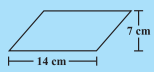1.Area of above shape =  =Area of above shape =Area of above shape =Area of above triangle =Area of above shape = b*h =

Question:(b) Write the perimeter of each shape.Perimeter of shape =perimeter of shape =perimeter of shape =perimeter of shape =perimeter of this shape cannot be calculated only with height and breadth,we need slant height or angle.

## Solutions of NCERT for class 8 maths chapter 11 mensuration-Exercise: 11.1Given:

Perimeter of square = perimeter of rectangle

Area of square

Area of rectangle =

Hence, area of square is greater than area of rectangle.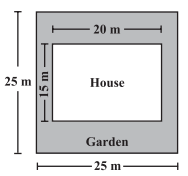Area of plot =

Area of house =

Area of garden around house =

the rate = 55 per .

the total cost of developing a garden around the house=Length of rectangle is 20 – (3.5 + 3.5) metres=13 metres

Breadth of rectangle = 7 metres

diameter of circular side = 7metres

Area of garden = area of rectangle + 2 times area of semi circular part

Area of garden

Perimetre of garden

metres

Area of parallelogram

a floor of area  =  =

Number of tiles =  =  tiles

Remember, circumference of a circle can be obtained by using the expression , where r is the radius of the circle.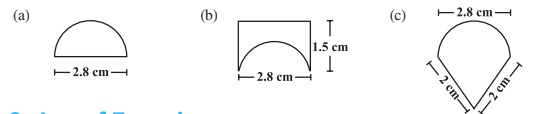(a) circumference

(b)circumference

(c) circumference

Hence, the perimeter of (b) is highest so ant have to take a longer round in (b).

## Question:1  Nazma’s sister also has a trapezium-shaped plot. Divide it into three parts as shown (Fig 11.4). Show that the area of a trapezium

.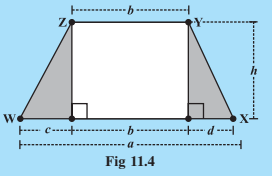Area of trapezium WXYZ = Area of triangle with base 'c' + area of rectangle + area of triangle with base'd'

Taking 'h' common, we get

Replacing

Hence proved that the area of a trapeziumArea of trapezium WXYZ = Area of traingle with base'c'+area of rectangle +area of triangle with base 'd'

the area WXYZ by  the expression

.

Hence,we can conclude area from given expression and calculated area is equal.

## Solutions of NCERT for class 8 maths chapter 11 mensuration topic 11.3 area of trapezium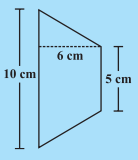(i) Area of trapezium(ii)Area of trapezium

## Solutions of NCERT for class 8 maths chapter 11 mensuration topic 11.4 area of a general quadrilateral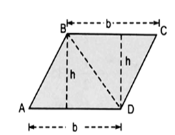This agree with the formula that we know already.

NCERT solutions for class 8 maths chapter 11 mensuration topic 11.4.1 area of a special quadrilaterals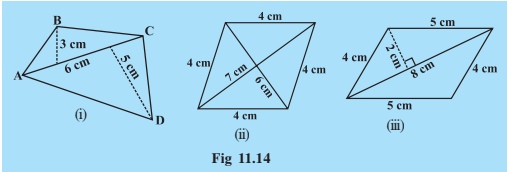(i) Area=

(ii) Area=

(iii)Area=

Solutions of NCERT for class 8 maths chapter 11 mensuration topic 11.5 area of a polygonFI is a diagonal of polygon EFGHI         NQ is a diagonal of polygon MNOPQR

(i)Area of  polygon EFGHI = area of EFI + area of quadrilateral FGHI

Draw a diagonal FH

Area of  polygon EFGHI = area of EFI + area of  FGH + area of FHIArea of polygon MNOPQR = area of quadrilateral NMRQ+area of quadrilateral NOPQ

Draw diagonal NP and NR .

Area of polygon MNOPQR = area of NOP+area  of NPQ +area of NMR +area of  NRQthe area of polygon MNOPQR

= area of MAN + area of trapezium ACON+area of  CPO + area of  MBR+area of trapezium BDQR+area of  DPQ

NCERT solutions for class 8 maths chapter 11 mensuration-Exercise: 11.2Area of table =

Let the length of the other parallel side be x

area of a trapezium =

cm

Hence,the length of the other parallel side is 7cm, and ,

Length of the fence of a trapezium shaped field  =  =

Area of trapezium =The diagonal of a quadrilateral shaped field is 24 m

the perpendiculars are 8 m and 13 m.

the area of the field =

Area of rhombus =

Rhombus is a type of parallelogram and area of parallelogram is product of base and height.

So,Area of rhombus = base  height

Let the other diagonal be x

Area of rhombus =

Area of 1 tile =

Area of 3000 tiles =

Total cost of polishing =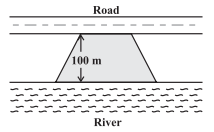Let the length of the side along road be x m.

Then according to question, lenght of side along river will be 2x m.

So equation becomes :

or

or

So the length of the side along the river is 2x = 140m.Area of octagonal surface = Area of rectangular surface + 2(Area of trapezium surface)

Area of recatangular surface =

Area of trapezium surface =

So total area of octagonal surface = 55 + 2(32) = 55 + 64 = 119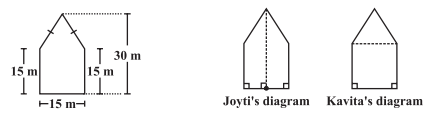Find the area of this park using both ways. Can you suggest some other way of finding its area?

Area of pentagonal park according to Jyoti's diagram :-

= 2(Area of trapezium)   =

Area of pentagonal park according to Kavita's diagram :-

= Area of triangle + Area of square.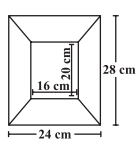Area of opposite sections will be same.

So area of horizontal sections,

And area of vertical sections,

Solutions of NCERT for class 8 maths chapter 11 mensuration topic 11.7.1 cuboid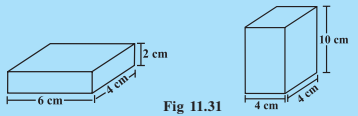(i)  Total surface area = 2 (h × l + b × h + b × l) = 2(lb + bh + hl)

(ii) Total surface area = 2 (h × l + b × h + b × l) = 2(lb + bh + hl)

CBSE NCERT solutions for class 8 maths chapter 11 mensuration topic 11.7.2 cubeSurface area of cube A =

=

Lateral surface area of cube B =

NCERT solutions for class 8 maths chapter 11 mensuration topic 11.7.3 cylinders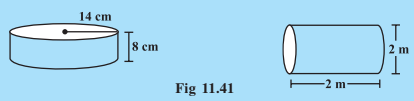Total surface area of cylinder = 2πr (r + h)

(i)  Area =

(ii) Area =

Solutions of NCERT for class 8 maths chapter 11 mensuration-Exercise: 11.3Surface area of cuboid (a)

Surface area of cube (b)

So box (a) requires the lesser amount of material to make.

Surface area of suitcase    .

Area of such 100 suitcase will be

So lenght of tarpaulin cloth

Surface area of cube

So,

or

or                           .

Thus side of cube is 10 cm.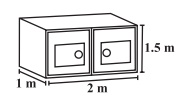Required area = Total area - Area of bottom surface

Total area

Area of bottom surface

So required area =

Total area painted by Daniel :

(  Bottom surface is excluded.)

So,  Area

No. of cans of paint required

Thus 5 cans of paint are required.The two figures have same height.The diference between them is one is cylinder and another is cube.

lateral surface area of cylinder =

lateral surface area of cube =

Cube  has a larger lateral surface area.

Total surface area of cylinder = 2πr (h + r)

Lenght of rectangular sheet

So perimeter of rectangular sheet = 2(l + b)

.

Thus perimeter of rectangular sheet is 322 cm.Area in one complete revolution of roller =  2πrh.Area of label =  2πrh

Here height is found out as = 20-2-2 = 16 cm.

CBSE NCERT solutions for class 8 maths chapter 11 mensuration topic 11.8.1 cuboid

Question:1 Find the volume of the following cuboids(i) Volume of cuboid is given as:

,

So, Given that Length = 8cm, Breadth = 3cm, and height = 2 cm so,

its volume will be = .

Aslo for Given Surface area of cuboid  and height = 3 cm we can easily calculate the volume:

;

So, Volume =

Solutions of NCERT for class 8 maths chapter 11 mensuration topic 11.8.2 cube

(a) with a side 4 cm             (b) with a side 1.5 m

(a) Volume of cube having side equal to 4cm will be

or    .

(b) When having side length equal to 1.5m then ,

.

Solutions of NCERT for class 8 maths chapter 11 mensuration topic 11.8.3 cylinder(i) The volume of a cylinder given as =  .

or given radius of cylinder = 7cm and length of cylinder = 10cm.

So, we can calculate the volume of the cylinder =           (Take the value of  )

The volume of cylinder = .

(ii) Given for the Surface area =  and height = 2m .

we have .

Solutions for NCERT class 8 maths chapter 11 mensuration-Exercise: 11.4

To find how much it can hold.

(a) To find out how much the cylindrical tank can hold we will basically find out the volume of the cylinder.

Number of cement bags required to plaster it.

(b) if we want to find out the cement bags required to plaster it means the area to be applied, we then calculate the surface area of the bags.Given the diameter of cylinder A = 7cm and the height = 14cm.

Also, the diameter of cylinder B = 14cm and height = 7cm.

We can easily suggest whose volume is greater without doing any calculations:

As volume is directly proportional to the square of the radius of cylinder and directly proportional to the height of the cylinder hence

B has more Volume as compared to A because B has a larger diameter.

Verifying:

Volume of A :

and Volume of B :  .

Hence clearly we can see that the volume of cylinder B is greater than the volume of cylinder A.

The cylinder B has surface area of  = .

and the surface area of cylinder A = .

The cylinder with greater volume also has greater surface area.

Given that the height of a cuboid whose base area is  and volume is ;

As

So, we have relation:

or, Height = 5cm.

So given the dimensions of cuboid  hence it's the volume is equal to =

We have to make small cubes with side 6cm which occupies the volume  =

Hence we have now one cube having side length = 6cm volume = .

So, total numbers of small cubes that can be placed in the given cuboid =

Hence 450 small cubes can be placed in that cuboid.

Given that the volume of the cylinder is  and having its diameter of base = 140cm.

So, as ;

hence putting in the relation we get;

The height of the cylinder would be = .

Volume of the cylinder = V =

So the quantity of milk in litres that can be stored in the tank is 49500 litres.

Question:7(i) If each edge of a cube is doubled,

how many times will its surface area increase?

The surface area of cube  =

So if we double the edge l becomes 2l.

New surface area =

Thus surface area becomes 4 times.

Question:7(ii) If each edge of a cube is doubled,

how many times will its volume increase?

Volume of cube =

Since l becomes 2l, so new volume is :     .

Hence volume becomes 8 times.Given that the water is pouring into a cuboidal reservoir at the rate of 60 litres per minute.

Volume of the reservoir is , then

The number of hours it will take to fill the reservoir will be:

As we know .

Then  = 108,000 Litres ;

Time taken to fill the tank will be:

or   .

## NCERT solutions for class 8 maths: Chapter-wise

 Chapter -1 NCERT solutions for class 8 maths chapter 1 Rational Numbers Chapter -2 Solutions of NCERT for class 8 maths chapter 2 Linear Equations in One Variable Chapter-3 CBSE NCERT solutions for class 8 maths chapter 3 Understanding Quadrilaterals Chapter-4 NCERT solutions for class 8 maths chapter 4 Practical Geometry Chapter-5 Solutions of NCERT for class 8 maths chapter 5 Data Handling Chapter-6 CBSE NCERT solutions for class 8 maths chapter 6 Squares and Square Roots Chapter-7 NCERT solutions for class 8 maths chapter 7 Cubes and Cube Roots Chapter-8 Solutions of NCERT for class 8 maths chapter 8 Comparing Quantities Chapter-9 NCERT solutions for class 8 maths chapter 9 Algebraic Expressions and Identities Chapter-10 CBSE NCERT solutions for class 8 maths chapter 10 Visualizing Solid Shapes Chapter-11 NCERT solutions for class 8 maths chapter 11 Mensuration Chapter-12 Solutions of NCERT for class 8 maths chapter 12 Exponents and Powers Chapter-13 CBSE NCERT solutions for class 8 maths chapter 13 Direct and Inverse Proportions Chapter-14 NCERT solutions for class 8 maths chapter 14 Factorization Chapter-15 Solutions of NCERT for class 8 maths chapter 15 Introduction to Graphs Chapter-16 CBSE NCERT solutions for class 8 maths chapter 16 Playing with Numbers

## NCERT solutions for class 8: Subject-wise

Some important formulas from NCERT solutions for class 8 maths chapter 11 mensuration to remember-

• Area of a trapezium

• Area of a rhombus

• The surface area of a cuboid

l- length of the cuboid

h- height of the cuboid

• The surface area of a cube

• The Surface area of a cylinder

h- height of the cylinder

• The volume of a cuboid

l- length of the cuboid

h- height of the cuboid

• The volume of a cube = l3
• The volume of a cylinder = πr2h

h- height of the cylinder

Tip- If you have derived the formulas and know how to drive it, then you can solve any problem of this chapter easily. You can take help from NCERT solutions for class 8 maths chapter 11 mensuration if you are not able to solve the problem. It will make your task easy.